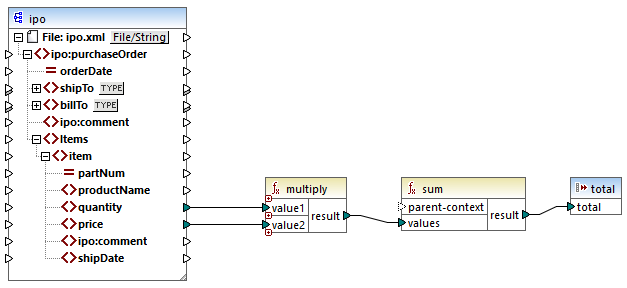# Altova MapForce 2022 Enterprise Edition

## core | aggregate functions

"Aggregating" means processing multiple values of the same type so as to obtain a single result, such as a sum, a count, or an average. You can perform data aggregation in MapForce with the help of aggregation functions, such as avg, count, max, and others.

The following two arguments are common to all aggregation functions:

1.parent-context. This argument is optional; it lets you override the default mapping context (and thus change the scope of the function, or the values that the function must iterate over). For a worked example, see Example: Changing the Parent Context.

2.values. This argument must be connected to a source item that supplies the values to be processed. For example, in the mapping illustrated below, the sum function takes as input a sequence of numeric values that originates from a source XML file. For each item in the source XML file, the multiply function gets the item's price times quantity, and passes the result to the sum function. The sum function will aggregate all input values and produce a total result that is also the output of the mapping. You can find this mapping in the MapForceExamples folder.SimpleTotal.mfd

Some aggregate functions, such as min, max, sum, and avg, work exclusively with numeric values. The input data of these functions is converted to the decimal data type for processing.